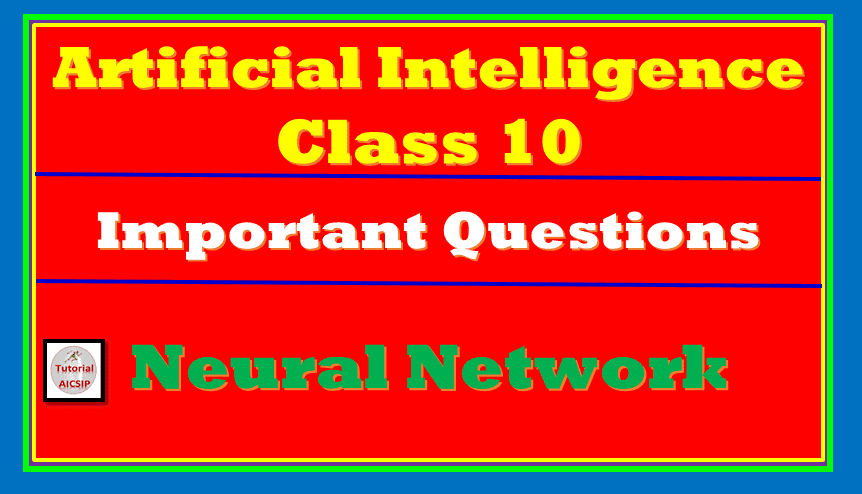In this article, we are going to discuss Important QnA for Neural Network AI class 10. This article will help students of Artificial Intelligence Class 9 and Artificial Intelligence Class 10 students. Here I am going to provide you questions for Artificial Intelligence class 9 and Class 10 Solutions. So let’s we start!

Topics Covered

## Important QnA Neural Network AI Class 10

This article, Important QnA Neural Network AI Class 10 will start with objective-type questions. So here we go!

## Objective type questions (OTQs)

1. A ________ is the technique to create computer programs to learn from data and aimed to functions like the human brain. (Ans. neural network)
2. The neural network is also known as _______. (Ans. Artificial Neural Network)
3. Which of the following is the feature of a neural network?
• Extract information without any programming
• Provide the filtered information without input
• Learn itself and produced the trained data
• All of these
4. Neural networks learn from the processing numerous _________________. (Ans. labeled examples)
5. The labeled examples are provided in the process of training. (True/False)
6. The traditional Machine Learning algorithm cannot improve the performance of the model after certain levels and then saturation will start. (True/False)
7. Large ANN performs better than as Medium and Small Neural Network datasets. (True/False)
8. The first layer of the neural network is known as __________ layer. (Ans. input)
9. The actual processing occurs in which of the following layer?
• input layers
• hidden layers
• output layers
• None of these
10. A neural network is used to solve problems using a large data set. (True/False)
11. In which of the following type of learning networks are trained to provide the correct output using several example units?
• Supervised learning
• Unsupervised learning
• Reinforcement learning
• All of these
12. In _________ learning only inputs provided but no output related data. (Ans. Unsupervised)
13. Reinforcement learning relies on output-related information. (True/False)

Now in the next section of neural network AI class 10 we are going to discuss subjective type questions.

Watch this video for more understanding:

## Subjective Type Questions (STQs)

In this section, we will talk about subjective type questions on the topic Neural Network AI class 10. These questions can be asked in 2 marks or 4 marks questions.

1. What do you mean by the neural network?
• The neural network is a model that works as neurons works in human brains.
• It is also known as ANN (Artificial Neural Networks)
• It copies the mechanism as working in human brains.
• It can extract the information without any code or programming.
• In a neural network, the machine can learn, recognize and make decisions like human beings.
2. Where the neural network used?
• A neural network is used in many fields where a large data set is required..
• It can be used in the following:
• Voice Recognition
• Character Recognition
• Signature Verification Applications
• Human face recognition
3. How does the neural network work?
• The input layers inputs or fed data
• The hidden layers process these data and assign weight to each layer randomly
• Every layer process has its own block which accomplishes the task and passes to the next layer.
• Then it comes to the output layer where machine learning executes the data received from input layers.
• Finally, the processed data passed to the output layer.
4. What are the features of a neural network?
• The model of the neural network follows the mechanism of the human brain
• It extracts information without any input from the user
• It basically works on a mathematical way with machine algorithms
• Suitable for large datasets
• The top layers are input layers to fed data, hidden layers process the data, and the output layer generates output from the processed data
5. Compare the three types of neural network learning.
• The three types of neural network learning are as following:

That’s all from the QnA session of Neural Network AI Class 10. If you have query regarding this topic Neural Network AI Class 10, feel free to ask in the comment section.